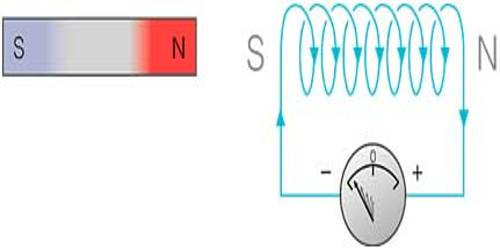# Lenz’s Law of Electromagnetic Induction

Lenz’s Law of Electromagnetic Induction

Faraday knew how to determine the direction of an induced current, but scientist Lenz gave a law for the determination of the direction of induced current due to the electromotive force and gave an appropriate explanation of it. This law is known as Lenz’s law of electromagnetic induction. The law is stated below:

Lenz’s law: In case of electromagnetic induction, the direction of the induced current (or emf) is such as to oppose the change of magnetic flux which causes the production of induced current (or emf) in the circuit.

In other words, the direction of induced current is such as to oppose the very cause which is responsible for inducing the current (or emf) in the circuit.

So Lenz’s law of electromagnetic induction states that, when an emf is induced according to Faraday’s law, the polarity (direction) of that induced emf is such that it opposes the cause of its production. Lenz’s law is based on Faraday’s law of induction, so before understanding Lenz’s law; one should know what is Faraday’s law of induction.

Thus, considering Lenz’s law

E = -N (dΦ/dt)   (volts)

The negative sign shows that the direction of the induced emf and the direction of change in magnetic fields have opposite signs.